Balbharti 12th Maharashtra State Board Maths Solutions Book Pdf Chapter 6 Line and Plane Miscellaneous Exercise 6B Questions and Answers.

## Maharashtra State Board 12th Maths Solutions Chapter 6 Line and Plane Miscellaneous Exercise 6B

Question 1.
If the line $$\frac{x}{3}=\frac{y}{4}$$ = z is perpendicular to the line $$\frac{x-1}{k}=\frac{y+2}{3}=\frac{z-3}{k-1}$$ then the value of k is: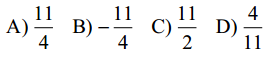Solution:
(b) $$-\frac{11}{4}$$

Question 2.
The vector equation of line 2x – 1 = 3y + 2 = z – 2 is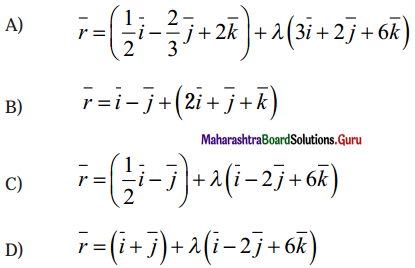Solution:
(a) $$\bar{r}=\left(\frac{1}{2} \hat{i}-\frac{2}{3} \hat{j}+2 \hat{k}\right)+\lambda(3 \hat{i}+2 \hat{j}+6 \hat{k})$$

Question 3.
The direction ratios of the line which is perpendicular to the two lines $$\frac{x-7}{2}=\frac{y+17}{-3}=\frac{z-6}{1}$$ and $$\frac{x+5}{1}=\frac{y+3}{2}=\frac{z-6}{-2}$$ are
(A) 4, 5, 7
(B) 4, -5, 7
(C) 4, -5, -7
(D) -4, 5, 8
Solution:
(A) 4, 5, 7Question 4.
The length of the perpendicular from (1, 6, 3) to the line $$\frac{x}{1}=\frac{y-1}{2}=\frac{z-2}{3}$$
(A) 3
(B) $$\sqrt {11}$$
(C) $$\sqrt {13}$$
(D) 5
Solution:
(C ) $$\sqrt {13}$$

Question 5.
The shortest distance between the lines $$\bar{r}=(\hat{i}+2 \hat{j}+\hat{k})+\lambda(\hat{i}-\hat{j}-\hat{k})$$ and $$\bar{r}=(2 \hat{i}-\hat{j}-\hat{k})+\mu(2 \hat{i}+\hat{j}+2 \hat{k})$$ is
Question is modified.
The shortest distance between the lines $$\bar{r}=(\hat{i}+2 \hat{j}+\hat{k})+\lambda(\hat{i}-\hat{j}+\hat{k})$$ and $$\bar{r}=(2 \hat{i}-\hat{j}-\hat{k})+\mu(2 \hat{i}+\hat{j}+2 \hat{k})$$ is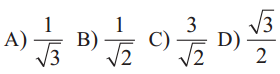Solution:
(c) $$\frac{3}{\sqrt{2}}$$

Question 6.
The lines $$\frac{x-2}{1}=\frac{y-3}{1}=\frac{z-4}{-k}$$ and $$\frac{x-1}{k}=\frac{y-4}{2}=\frac{z-5}{1}$$. and coplanar if
(A) k = 1 or -1
(B) k = 0 or -3
(C) k = + 3
(D) k = 0 or -1
Solution:
(B ) k = 0 or -3

Question 7.
The lines $$\frac{x}{1}=\frac{y}{2}=\frac{z}{3}$$ and $$\frac{x-1}{-2}=\frac{y-2}{-4}=\frac{z-3}{6}$$ and are
(A) perpendicular
(B) inrersecting
(C) skew
(D) coincident
Solution:
(B) inrersectingQuestion 8.
Equation of X-axis is
(A) x = y = z
(B) y = z
(C) y = 0, z = 0
(D) x = 0, y = 0
Solution:
(C) y = 0, z = 0

Question 9.
The angle between the lines 2x = 3y = -z and 6x = -y = -4z is
(A ) 45º
(B ) 30º
(C ) 0º
(D ) 90º
Solution:
(D ) 90º

Question 10.
The direction ratios of the line 3x + 1 = 6y – 2 = 1 – z are
(A ) 2, 1, 6
(B ) 2, 1, -6
(C ) 2, -1, 6
(D ) -2, 1, 6
Solution:
(B ) 2, 1, -6Question 11.
The perpendicular distance of the plane 2x + 3y – z = k from the origin is $$\sqrt {14}$$ units, the value
of k is
(A ) 14
(B ) 196
(C ) $$2\sqrt {14}$$
(D ) $$\frac{\sqrt{14}}{2}$$
Solution:
(A ) 14

Question 12.
The angle between the planes and $$\bar{r} \cdot(\bar{i}-2 \bar{j}+3 \bar{k})+4=0$$ and $$\bar{r} \cdot(2 \bar{i}+\bar{j}-3 \bar{k})+7=0$$ is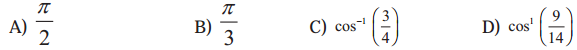Solution:
(d) cos-1$$\left(\frac{9}{14}\right)$$

Question 13.
If the planes $$\bar{r} \cdot(2 \bar{i}-\lambda \bar{j}+\bar{k})=3$$ and $$\bar{r} \cdot(4 \bar{i}-\bar{j}+\mu \bar{k})=5$$ are parallel, then the values of λ and μ are respectively.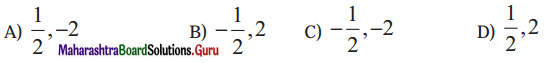Solution:
(d) $$\frac{1}{2}$$, 2

Question 14.
The equation of the plane passing through (2, -1, 3) and making equal intercepts on the coordinate axes is
(A ) x + y + z =1
(B ) x + y + z = 2
(C ) x + y + z = 3
(D ) x + y + z = 4
Solution:
(D ) x + y + z = 4Question 15.
Measure of angle between the planes 5x – 2y + 3z – 7 = 0 and 15x – 6y + 9z + 5 = 0 is
(A ) 0º
(B ) 30º
(C ) 45º
(D ) 90º
Solution:
(A ) 0º

Question 16.
The direction cosines of the normal to the plane 2x – y + 2z = 3 are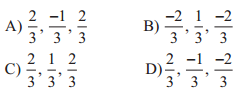Solution:
(a) $$\frac{2}{3}, \frac{-1}{3}, \frac{2}{3}$$

Question 17.
The equation of the plane passing through the points (1, -1, 1), (3, 2, 4) and parallel to Y-axis is :
(A ) 3x + 2z – 1 = 0
(B ) 3x – 2z = 1
(C ) 3x + 2z + 1 = 0
(D ) 3x + 2z = 2
Solution:
(B ) 3x – 2z = 1

Question 18.
The equation of the plane in which the line $$\frac{x-5}{4}=\frac{y-7}{4}=\frac{z+3}{-5}$$ and $$\frac{x-8}{7}=\frac{y-4}{1}=\frac{z+5}{3}$$ lie, is
(A ) 17x – 47y – 24z + 172 = 0
(B ) 17x + 47y – 24z + 172 = 0
(C ) 17x + 47y + 24z +172 = 0
(D ) 17x – 47y + 24z + 172 = 0
Solution:
(A ) 17x – 47y – 24z + 172 = 0Question 19.
If the line $$\frac{x+1}{2}=\frac{y-m}{3}=\frac{z-4}{6}$$ lies in the plane 3x – 14y + 6z + 49 = 0, then the value of m is:
(A ) 5
(B ) 3
(C ) 2
(D ) -5
Solution:
(A ) 5

Question 20.
The foot of perpendicular drawn from the point (0,0,0) to the plane is (4, -2, -5) then the equation of the plane is
(A ) 4x + y + 5z = 14
(B ) 4x – 2y – 5z = 45
(C ) x – 2y – 5z = 10
(D ) 4x + y + 6z = 11
Solution:
(B ) 4x – 2y – 5z = 45

II. Solve the following :
Question 1.
Find the vector equation of the plane which is at a distance of 5 unit from the origin and which is normal to the vector $$2 \hat{i}+\hat{j}+2 \hat{k}$$
Solution:
If $$\hat{n}$$ is a unit vector along the normal and p i the length of the perpendicular from origin to the plane, then the vector equation of the plane $$\bar{r} \cdot \hat{n}$$ = p
Here, $$\overline{\mathrm{n}}=2 \hat{\mathrm{i}}+\hat{\mathrm{j}}+2 \hat{\mathrm{k}}$$ and p = 5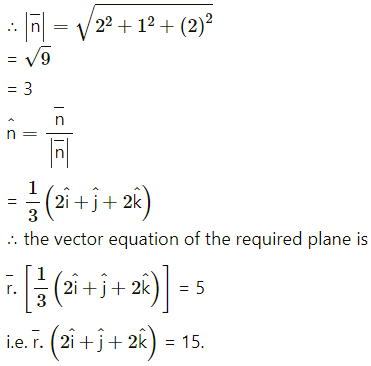Question 2.
Find the perpendicular distance of the origin from the plane 6x + 2y + 3z – 7 = 0
Solution:
The distance of the point (x1, y1, z1) from the plane ax + by + cz + d is $$\left|\frac{a x_{1}+b y_{1}+c z_{1}+d}{\sqrt{a^{2}+b^{2}+c^{2}}}\right|$$
∴ the distance of the point (1, 1, -1) from the plane 6x + 2y + 3z – 7 = 0 is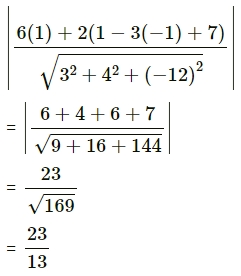= 1units.

Question 3.
Find the coordinates of the foot of the perpendicular drawn from the origin to the plane 2x + 3y + 6z = 49.
Solution:
The equation of the plane is 2x + 3y + 6z = 49
Dividing each term by
$$\sqrt{2^{2}+3^{2}+(-6)^{2}}$$
= $$\sqrt{49}$$
= 7
we get
$$\frac{2}{7}$$x + $$\frac{3}{7}$$y – $$\frac{6}{7}$$z = $$\frac{49}{7}$$ = 7
This is the normal form of the equation of plane.
∴ the direction cosines of the perpendicular drawn from the origin to the plane are
l = $$\frac{2}{7}$$, m = $$\frac{3}{7}$$, n = $$\frac{6}{7}$$
and length of perpendicular from origin to the plane is p = 7.
the coordinates of the foot of the perpendicular from the origin to the plane are
(lp, ∓, np)i.e.(2, 3, 6)

Question 4.
Reduce the equation $$\bar{r} \cdot(\hat{i}+8 \hat{j}+24 \hat{k})=13$$ to normal form and hence find
(i) the length of the perpendicular from the origin to the plane
(ii) direction cosines of the normal.
Solution:
The normal form of equation of a plane is $$\bar{r} \cdot \hat{n}$$ = p where $$\hat{n}$$ is unit vector along the normal and p is the length of perpendicular drawn from origin to the plane.
Given pane is $$\text { r. }(6 \hat{\mathrm{i}}+8 \hat{\mathrm{j}}+24 \hat{\mathrm{k}})=13$$ …(1)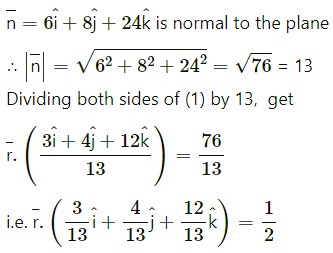This is the normal form of the equation of plane.
Comparing with $$\bar{r} \cdot \hat{n}$$ = p,
(i) the length of the perpendicular from the origin to plane is $$\frac{1}{2}$$.
(ii) direction cosines of the normal are $$\frac{3}{13}, \frac{4}{13}, \frac{12}{13}$$Question 5.
Find the vector equation of the plane passing through the points A(1, -2, 1), B (2, -1, -3) and C (0, 1, 5).
Solution:
The vector equation of the plane passing through three non-collinear points A($$\bar{a}$$), B($$\bar{b}$$) and C($$\bar{c}$$) is $$\bar{r} \cdot(\overline{\mathrm{AB}} \times \overline{\mathrm{AC}})=\bar{a} \cdot(\overline{\mathrm{AB}} \times \overline{\mathrm{AC}})$$ … (1)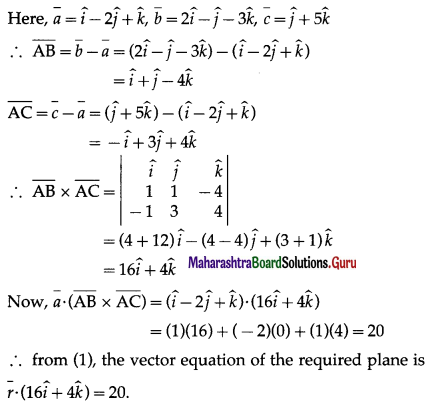Question 6.
Find the Cartesian equation of the plane passing through A(1, -2, 3) and the direction ratios of whose normal are 0, 2, 0.
Solution:
The Cartesian equation of the plane passing through (x1, y1, z1), the direction ratios of whose normal are a, b, c, is
a(x – x1) + b(y – y1) + c(z – z1) = 0
∴ the cartesian equation of the required plane is
o(x + 1) + 2(y + 2) + 5(z – 3) = 0
i.e. 0 + 2y – 4 + 10z – 15 = 0
i.e. y + 2 = 0.

Question 7.
Find the Cartesian equation of the plane passing through A(7, 8, 6) and parallel to the plane $$\bar{r} \cdot(6 \hat{i}+8 \hat{j}+7 \hat{k})=0$$
Solution:
The cartesian equation of the plane $$\bar{r} \cdot(6 \hat{i}+8 \hat{j}+7 \hat{k})=0$$ is 6x + 8y + 7z = 0 The required plane is parallel to it
∴ its cartesian equation is
6x + 8y + 7z = p …(1)
A (7, 8, 6) lies on it and hence satisfies its equation
∴ (6)(7) + (8)(8) + (7)(6) = p
i.e., p = 42 + 64 + 42 = 148.
∴ from (1), the cartesian equation of the required plane is 6x + 8y + 7z = 148.Question 8.
The foot of the perpendicular drawn from the origin to a plane is M(1, 2,0). Find the vector equation of the plane.
Solution:
The vector equation of the plane passing through A($$\bar{a}$$) and perpendicular to $$\bar{n}$$ is $$\bar{r} \cdot \bar{n}=\bar{a} \cdot \bar{n}$$.
M(1, 2, 0) is the foot of the perpendicular drawn from origin to the plane. Then the plane is passing through M and is
perpendicular to OM.
If $$\bar{m}$$ is the position vector of M, then $$\bar{m}$$ = $$\hat{\mathrm{i}}$$.
Normal to the plane is
$$\bar{n}$$ = $$\overline{\mathrm{OM}}$$ = $$\hat{\mathrm{i}}$$
$$\overline{\mathrm{m}} \cdot \overline{\mathrm{n}}$$ = $$\hat{\mathrm{i}}, \hat{i}$$ = 5
∴ the vector equation of the required plane is
$$\bar{r} \cdot(\hat{i}+2 \hat{j})$$ = 5

Question 9.
A plane makes non zero intercepts a, b, c on the co-ordinates axes. Show that the vector equation of the plane is $$\bar{r} \cdot(b c \hat{i}+c a \hat{j}+a b \hat{k})$$ = abc
Solution:
The vector equation of the plane passing through A($$\bar{a}$$), B($$\bar{b}$$).. C($$\bar{c}$$), where A, B, C are non collinear is
$$\overline{\mathrm{r}} \cdot(\overline{\mathrm{AB}} \times \overline{\mathrm{AC}})=\overline{\mathrm{a}} \cdot(\overline{\mathrm{AB}} \times \overline{\mathrm{AC}})$$ …(1)
The required plane makes intercepts 1, 1, 1 on the coordinate axes.
∴ it passes through the three non collinear points A = (1, 0, 0), B = (0, 1, 0), C = (0, , 1)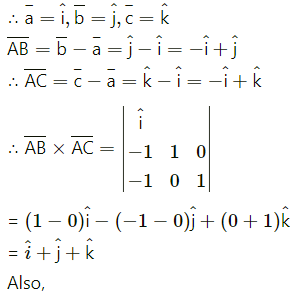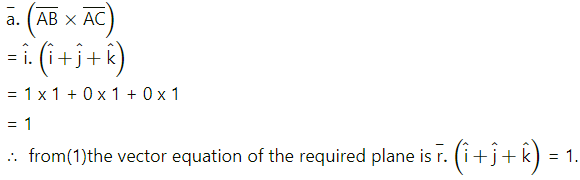Question 10.
Find the vector equation of the plane passing through the pointA(-2, 3, 5) and parallel to vectors $$4 \hat{i}+3 \hat{k}$$ and $$\hat{i}+\hat{j}$$
Solution:
The vector equation of the plane passing through the point A($$\bar{a}$$) and parallel to the vectors $$\bar{b}$$ and $$\bar{c}$$ is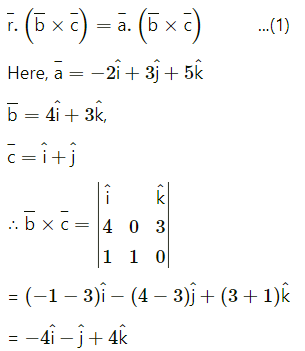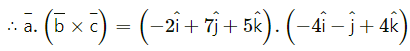= (-2)(-4) + (7)(-1) + (5)(4)
= 8 – 7 + 8
= 35
∴ From (1), the vector equation of the required plane is $$\overline{\mathrm{r}} \cdot(-3 \hat{\mathrm{i}}-3 a t \mathrm{j}+4 \hat{\mathrm{k}})$$ = 35.Question 11.
Find the Cartesian equation of the plane $$\bar{r}=\lambda(\hat{i}+\hat{j}-\hat{k})+\mu(\hat{i}+2 \hat{j}+3 \hat{k})$$
Solution:
The equation $$\bar{r}=\bar{a}+\lambda \bar{b}+\mu \bar{c}$$ represents a plane passing through a point having position vector $$\overline{\mathrm{a}}$$ and parallel to vectors $$\overline{\mathrm{b}}$$ and $$\overline{\mathrm{c}}$$.
Here,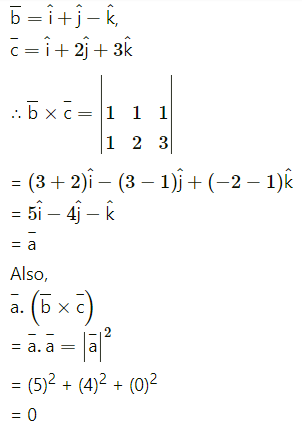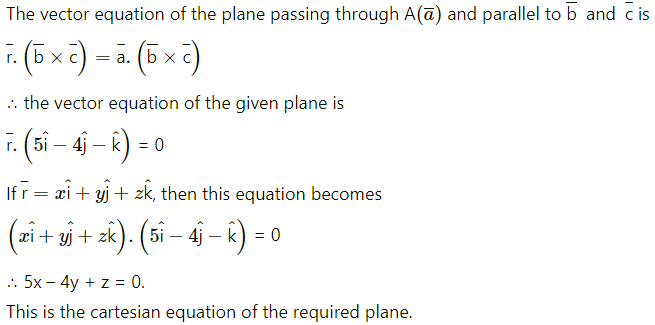Question 12.
Find the vector equations of planes which pass through A(1, 2, 3), B (3, 2, 1) and make equal intercepts on the co-ordinates axes.
Question is modified
Find the cartesian equations of the planes which pass through A(1, 2, 3), B(3, 2, 1) and make equal intercepts on the coordinate axes.
Solution:
Case 1 : Let all the intercepts be 0.
Then the plane passes through the origin.
Then the cartesian equation of the plane is
ax + by + cz = 0 …..(1)
(1, 2, 3) and (3, 2, 1) lie on the plane.
∴ a + 2b + 3c = 0 and 3a + 2b + c = 0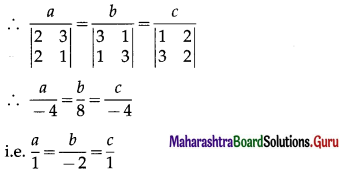∴ a, b, c are proportional to 1, -2, 1
∴ from (1), the required cartesian equation is x – 2y + z = 0.
Case 2 : Let the plane make non zero intercept p on each axis.
then its equation is $$\frac{x}{p}+\frac{y}{p}+\frac{z}{p}$$ = 1
i.e. x + y + z = p …(2)
Since this plane pass through (1, 2, 3) and (3, 2, 1)
∴ 1 + 2 + 3 = p and 3 + 2 + 1 = p
∴ p = 6
∴ from (2), the required cartesian equation is
x + y + z = 6
Hence, the cartesian equations of required planes are x + y + z = 6 and x – 2y + z = 0.Question 13.
Find the vector equation of the plane which makes equal non-zero intercepts on the co-ordinates axes and passes through (1, 1, 1).
Solution:
Case 1 : Let all the intercepts be 0.
Then the plane passes through the origin.
Then the vector equation of the plane is ax + by + cz …(1)
(1, 1, 1) lie on the plane.
∴ 1a + 1b + 1c = 0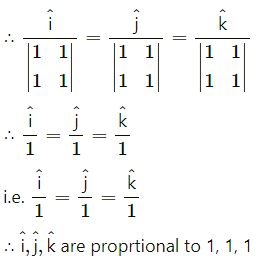∴ from (1), the required cartesian equation is x – y + z = 0
Case 2 : Let he plane make non zero intercept p on each axis.
then its equation is $$\frac{\hat{\mathrm{i}}}{p}+\frac{\hat{\mathrm{j}}}{p}+\frac{\hat{\mathrm{k}}}{p}=1$$ = 1
i.e. $$\hat{i}+\hat{j}+\hat{k}=p$$ = p ….(2)
Since this plane pass through (1, 1, 1)
∴ 1 + 1 + 1 = p
∴ p = 3
∴ from (2), the required cartesian equation is $$\hat{i}+\hat{j}+\hat{k}$$ = 3
Hence, the cartesian equations of required planes are $$\bar{r} \cdot(\hat{i}+\hat{j}+\hat{k})=3$$

Question 14.
Find the angle between planes $$\bar{r} \cdot(-2 \hat{i}+\hat{j}+2 \hat{k})=17$$ and $$\bar{r} \cdot(2 \hat{i}+2 \hat{j}+\hat{k})=71$$.
Solution:
The acute angle between the planes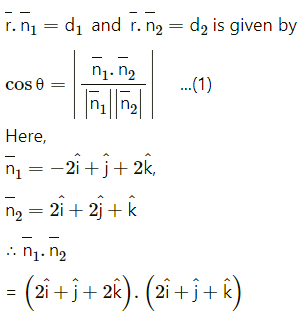= (1)(2) + (1)(1) + (2)(1)
= 2 + 1 + 2
= 5
Also,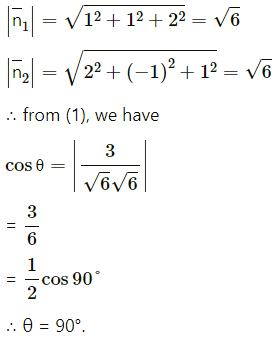Question 15.
Find the acute angle between the line $$\bar{r}=\lambda(\hat{i}-\hat{j}+\hat{k})$$ and the plane $$\bar{r} \cdot(2 \hat{i}-\hat{j}+\hat{k})=23$$
Solution:
The acute angle θ between the line $$\overline{\mathrm{r}}=\overline{\mathrm{a}}+\lambda \overline{\mathrm{b}}$$ and the plane $$\overline{\mathrm{r}} \cdot \overline{\mathrm{n}}$$ = d is given by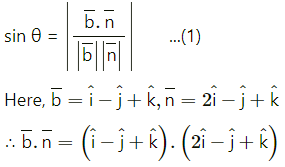= (2)(2) + (3)(-1) + (-6)(1)
= 4 – 3 – 6
= -5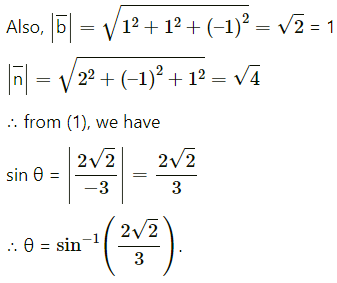Question 16.
Show that lines $$\bar{r}=(\hat{i}+4 \hat{j})+\lambda(\hat{i}+2 \hat{j}+3 \hat{k})$$ and $$\bar{r}=(3 \hat{j}-\hat{k})+\mu(2 \hat{i}+3 \hat{j}+4 \hat{k})$$
Solution:

Question 17.
Find the distance of the point $$3 \hat{i}+3 \hat{j}+\hat{k}$$ from the plane $$\bar{r} \cdot(2 \hat{i}+3 \hat{j}+6 \hat{k})=21$$
Solution:
The distance of the point A($$\bar{a}$$) from the plane $$\bar{r} \cdot \bar{n}$$ = p is given by d = $$\frac{|\bar{a} \cdot \bar{n}-p|}{|\bar{n}|}$$ ……(1)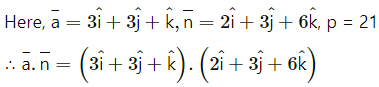= (3)(2) + (3)(3) + (1)(-6)
= 6 + 9 – 6
= 9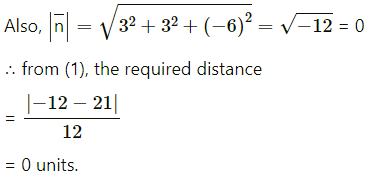Question 18.
Find the distance of the point (13, 13, -13) from the plane 3x + 4y – 12z = 0.
Solution: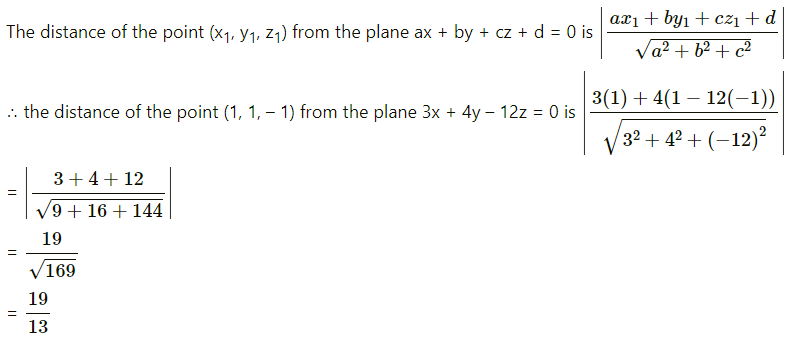= 19units.Question 19.
Find the vector equation of the plane passing through the origln and containing the line $$\bar{r}=(\hat{i}+4 \hat{j}+\hat{k})+\lambda(\hat{i}+2 \hat{j}+\hat{k})$$.
Solution:
The vector equation of the plane passing through A($$\bar{a}$$) and perpendicular to the vector $$\bar{n}$$ is $$\bar{r} \cdot \bar{n}=\bar{a} \cdot \bar{n}$$ … (1)
We can take $$\bar{a}$$ = $$\bar{0}$$ since the plane passes through the origin.
The point M with position vector $$\bar{m}$$ =$$\hat{i}+4 \hat{j}+\hat{k}$$ lies on the line and hence it lies on the plane.
.’. $$\overline{\mathrm{OM}}=\bar{m}=\hat{i}+4 \hat{j}+\hat{k}$$ lies on the plane.
The plane contains the given line which is parallel to $$\bar{b}=\hat{i}+2 \hat{j}+\hat{k}$$
Let $$\bar{n}$$ be normal to the plane. Then $$\bar{n}$$ is perpendicular to $$\overline{\mathrm{OM}}$$ as well as $$\bar{b}$$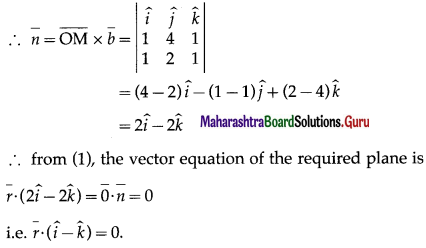Question 20.
Find the vector equation of the plane which bisects the segment joining A(2, 3, 6) and B( 4, 3, -2) at right angle.
Solution:
The vector equation of the plane passing through A($$\bar{a}$$) and perpendicular to the vector $$\bar{n}$$ is $$\bar{r} \cdot \bar{n}=\bar{a} \cdot \bar{n}$$ ….(1)
The position vectors $$\bar{a}$$ and $$\bar{b}$$ of the given points A and B are $$\bar{a}=2 \hat{i}+3 \hat{j}+6 \hat{k}$$ and $$\bar{b}=4 \hat{i}+3 \hat{j}-2 \hat{k}$$
If M is the midpoint of segment AB, the position vector $$\bar{m}$$ of M is given by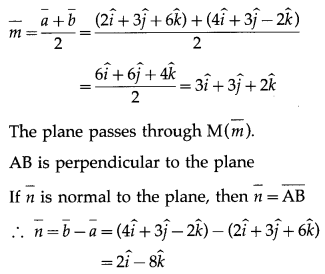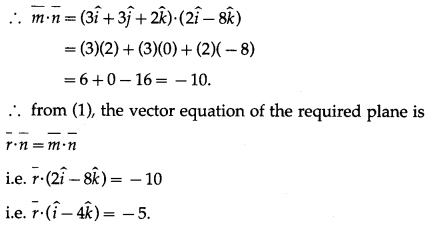Question 21.
Show thatlines x = y, z = 0 and x + y = 0, z = 0 intersect each other. Find the vector equation of the plane determined by them.
Solution:
Given lines are x = y, z = 0 and x + y = 0, z = 0.
It is clear that (0, 0, 0) satisfies both the equations.
∴ the lines intersect at O whose position vector is $$\overline{0}$$
Since z = 0 for both the lines, both the lines lie in XY- plane.
Hence, we have to find equation of XY-plane.
Z-axis is perpendicular to XY-plane.
∴ normal to XY plane is $$\hat{k}$$.
0($$\overline{0}$$) lies on the plane.
By using $$\bar{r} \cdot \bar{n}=\bar{a} \cdot \bar{n}$$, the vector equation of the required plane is $$\bar{r} \cdot \hat{k}=\overline{0} \cdot \bar{k}$$
i.e. $$\bar{r} \cdot \hat{k}=0$$.
Hence, the given lines intersect each other and the vector equation of the plane determine by them is $$\bar{r} \cdot \hat{k}=0$$.